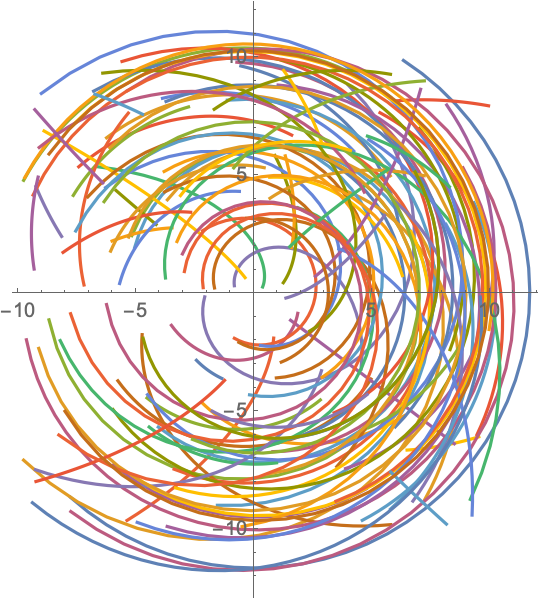#Function Repository Resource:

# PowerSubdivide

Subdivide an interval such that the ratio of subsequent elements is constant

Contributed by: Sander Huisman
 ResourceFunction["PowerSubdivide"][xmax,n] generates the list of values obtained by subdiving the interval from 1 to xmax into n parts such that the ratio of subsequent elements is constant. ResourceFunction["PowerSubdivide"][xmin,xmax,n] generates the list of values obtained by subdiving the interval from xmin to xmax into n parts such that the ratio of subsequent elements is constant.

## Details and Options

ResourceFunction["PowerSubdivide"] effectively behaves like Subdivide, but in “log-space”.
ResourceFunction["PowerSubdivide"][, n] generates a list of length n+1.

## Examples

### Basic Examples

Subdivide the range 10–10000 in three steps:

 In:=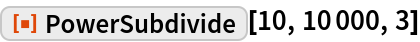Out=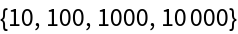### Scope

With two arguments, the start of the sequence is assumed to be 1:

 In:=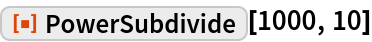Out=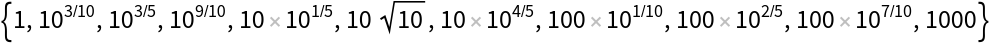PowerSubdivide can also perform on symbolic entries:

 In:=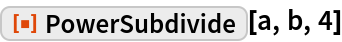Out=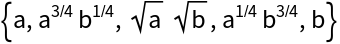xmin can be larger than xmax:

 In:=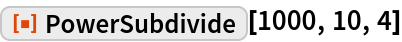Out=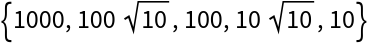### Applications

Choosing points logarithmically is useful for plotting on a logarithmic scale:

 In:=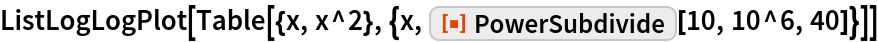Out=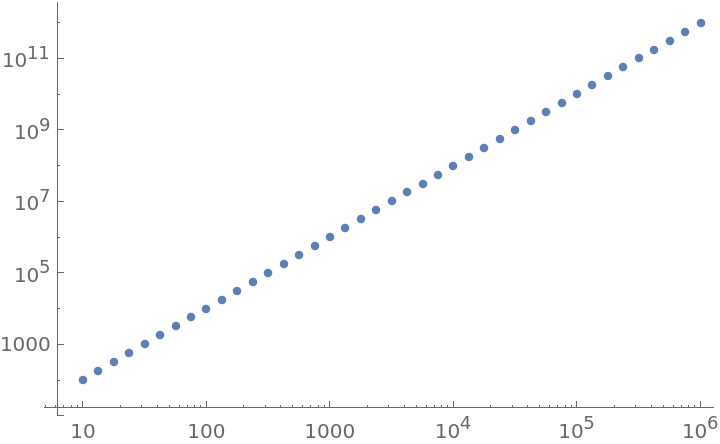### Properties and Relations

The ratio between subsequent values is constant:

 In:=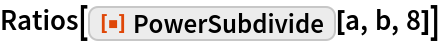Out=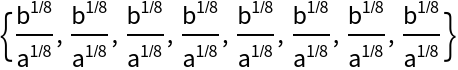PowerSubdivide is related to Subdivide:

 In:=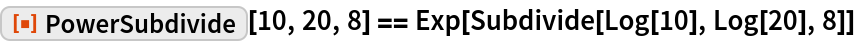Out=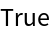Negative and positive end points result in intermediate values in the complex plane:

 In:=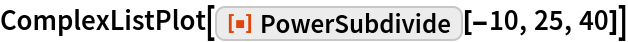Out=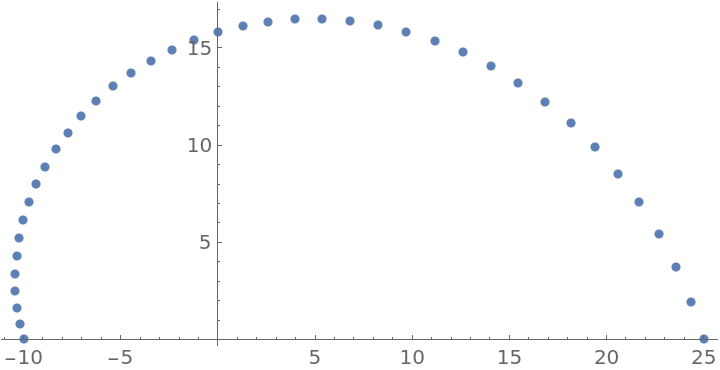Calculate the geometric mean of two values:

 In:=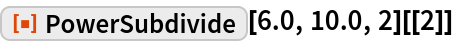Out=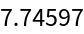Compare to the built-in function:

 In:=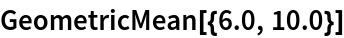Out=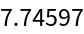### Possible Issues

If the start or end is a negative number, intermediate values might be complex numbers:

 In:=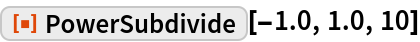Out=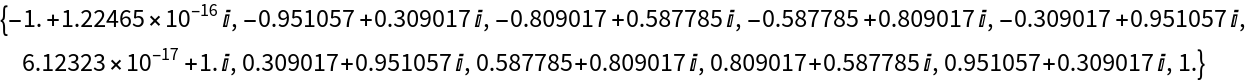If both end points are negative real numbers, Chop might be needed to remove approximate zeros:

 In:=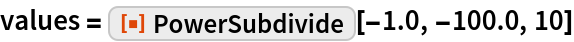Out=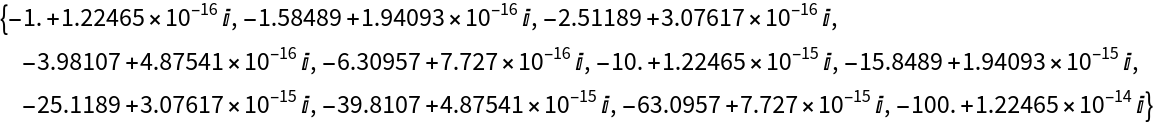In:=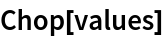Out=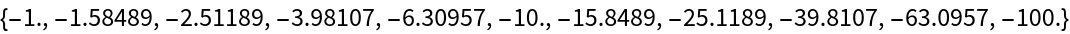Because Log equals -, all but the last entry will be 0:

 In:=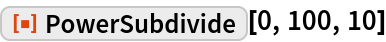Out=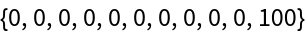### Neat Examples

Connect pairs of random complex numbers:

 In:=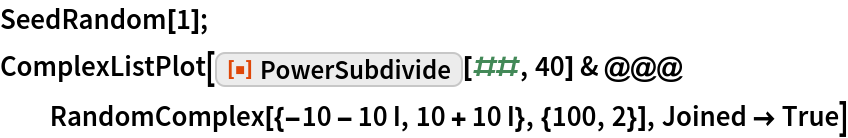Out=